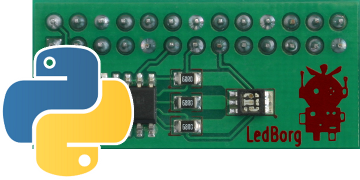# PyLed - Driving LedBorg directly using Python

Here is an example for everyone who wants to use an LedBorg without the driver code, or simply wants to see an example of GPIO used in PythonPyLed.py is a Python script which uses the RPi.GPIO library to set the state of the 3 GPIO pins representing the red, green and blue channels

Here's the code, you can download the PyLed script file as text here
Save the text file on your pi as PyLed.py
If you have the LedBorg driver installed you will need to temporarily disable it to use the GPIO pins instead using
`sudo /etc/init.d/ledborg.sh stop`
Make the script executable using
`chmod +x PyLed.py`
and run using
`sudo ./PyLed.py RGB`

```#!/usr/bin/env python
# coding: latin-1

# Import library functions we need
import sys
import RPi.GPIO as GPIO
GPIO.setmode(GPIO.BCM)
GPIO.setwarnings(False)

# Set which GPIO pins the LED outputs are connected to
LED_RED = 17
if GPIO.RPI_REVISION == 1:
LED_GREEN = 21                      # Rev 1 boards
else:
LED_GREEN = 27                      # Rev 2 boards
LED_BLUE = 22

# Set all of the LED pins as output pins
GPIO.setup(LED_RED, GPIO.OUT)
GPIO.setup(LED_GREEN, GPIO.OUT)
GPIO.setup(LED_BLUE, GPIO.OUT)

# Get the user input from the command or direct input
if len(sys.argv) > 1:
colour = sys.argv
else:
colour = raw_input('Colour number? ')

# Determine the pin levels
red = GPIO.LOW
green = GPIO.LOW
blue = GPIO.LOW
if len(colour) > 0:
if colour == '1' or colour == '2':
red = GPIO.HIGH
if len(colour) > 1:
if colour == '1' or colour == '2':
green = GPIO.HIGH
if len(colour) > 2:
if colour == '1' or colour == '2':
blue = GPIO.HIGH

# Apply the pin levels to the correct pins
GPIO.output(LED_RED, red)
GPIO.output(LED_GREEN, green)
GPIO.output(LED_BLUE, blue)
```